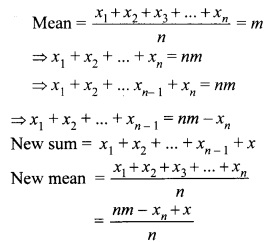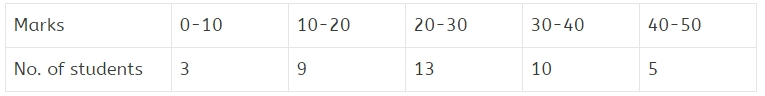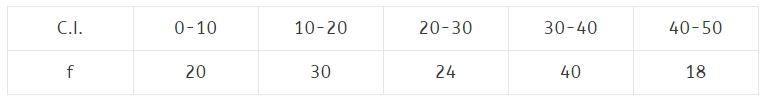# MCQ Questions for Class 10 Maths Chapter 14 Statistics with Answers

Check the below NCERT MCQ Questions for Class 10 Maths Chapter 14 Statistics with Answers Pdf free download. MCQ Questions for Class 10 Maths with Answers were prepared based on the latest exam pattern. We have provided Statistics Class 10 Maths MCQs Questions with Answers to help students understand the concept very well. https://mcqquestions.guru/mcq-questions-for-class-10-maths-chapter-14/

Students can also refer to NCERT Solutions for Class 10 Maths Chapter 14 Statistics for better exam preparation and score more marks.

## Statistics Class 10 MCQs Questions with Answers

Statistics Class 10 MCQ Question 1.
Cumulative frequency curve is also called
(a) histogram
(b) ogive
(c) bar graph
(d) median

Statistics MCQ Class 10 Question 2.
The relationship between mean, median and mode for a moderately skewed distribution is
(a) mode = median – 2 mean
(b) mode = 3 median – 2 mean
(c) mode = 2 median – 3 mean
(d) mode = median – mean

Answer: (b) mode = 3 median – 2 mean
Hint:
Mode = 3 median – 2 mean

MCQ On Statistics Class 10 Question 3.
The median of set of 9 distinct observations is 20.5. If each of the largest 4 observations of the set is increased by 2, then the median of the new set
(a) is increased by 2
(b) is decreased by 2
(c) is two times of the original number
(d) Remains the same as that of the original set.

Answer: (d) Remains the same as that of the original set.
Hint:
No. of observations = 9
∴ median = 5th observation
∵ The largest four observations are increased
∴ 5th observation remains unchanged.

Statistics Class 10 MCQ With Answers Question 4.
Mode and mean of a data are 12k and 15A. Median of the data is
(a) 12k
(b) 14k
(c) 15k
(d) 16k

Hint:
∵ Mode = 3 median – 2 mean
⇒ 12k = 3 median – 2 × 15k
⇒ 42k = 3 median
⇒ Median = 14k.

MCQ Of Statistics Class 10 Question 5.
The times, in seconds, taken by 150 atheletes to run a 110 m hurdle race are tabulated below:

 Class Frequency 13.8 – 14.0 2 14.0 – 14.2 4 14.2 – 14.4 5 14.4 – 14.6 71 14.6 – 14.8 48 14.8 – 15.0 20

The number of atheletes who completed the race in less then 14.6 seconds is:
(a) 11
(b) 71
(c) 82
(d) 130

Class 10 Statistics MCQ Question 6.
The abscissa of the point of intersection of the less than type and of the more than type cumulative frequency curves of a grouped data gives its
(a) mean
(b) median
(c) mode
(d) all the three above

Class 10 Maths Chapter 14 MCQ Question 7.
Mean of n numbers x1, x2, … xn is m. If xn is replaced by x, then new mean is
(a) m – xn + x
(b) $$\frac{nm-x_n+x}{n}$$
(c) $$\frac{(n-1)m+x}{n}$$
(d) $$\frac{m-x_n+x}{n}$$

Answer: (b) $$\frac{nm-x_n+x}{n}$$
Hint:Chapter 14 Maths Class 10 MCQ Question 8.
While computing mean of grouped data, we assume that the frequencies are [NCERT Exemplar Problems]
(a) evenly distributed over all the classes
(b) centred at the classmarks of the classes
(c) centred at the upper limits of the classes
(d) centred at the lower limits of the classes

Answer: (b) centred at the classmarks of the classes

Class 10 Maths Ch 14 MCQ Question 9.
Mean of 100 items is 49. It was discovered that three items which should have been 60, 70, 80 were wrongly read as 40, 20, 50 respectively. The correct mean is
(a) 48
(b) 49
(c) 50
(d) 60

Hint:
Sum of 100 observations
= 100 × 49 = 4900
Correct sum
= 4900 – [40 + 20 + 50 ] + [60 + 70 + 80] = 5000
∴ Correct mean = $$\frac{5000}{100}$$ = 50.

Ch 14 Maths Class 10 MCQ Question 10.
The times, in seconds, taken by 150 atheletes to run a 100 m hurdle race are tabulated below:

 Class Frequency 13.8-14 3 14 – 14.2 4 14.2 – 14.4 6 14.4 – 14.6 69 14.6 – 14.8 48 14.8 – 15.0 20

The number of atheletes who completed the race in less than 14.6 seconds is
(a) 13
(b) 69
(c) 82
(d) 130

MCQ Questions For Class 10 Maths Chapter 14 Question 11.
For the following distributionthe number of students who got marks less than 30 is
(a) 13
(b) 25
(c) 10
(d) 12

Chapter 14 Statistics MCQ Question 12.
In the given data:

 C.I Frequency 65-85 4 85 – 105 5 105 – 125 13 125 – 145 20 145 – 165 14 165 – 185 7 185 – 205 4

the difference of the upper limit of the median class and the lower limit of the modal class is
(a) 38
(b) 20
(c) 19
(d) 0

MCQ Questions For Class 10 Maths Statistics Question 13.
For the following distribution

 Marks No. of students Less than 20 4 Less than 40 12 Less than 60 25 Less than 80 56 Less than 100 74 Less than 120 80

the modal class is
(a) 20 – 40
(b) 40 – 60
(c) 60 – 80
(d) 80 -100

MCQs On Statistics Class 10 Question 14.
For the following distributionthe sum of lower limits of the modal class and the median class is
(a) 20
(b) 30
(c) 40
(d) 50

Class 10 Maths Statistics MCQs Question 15.
While computing mean of grouped data, we assume that the frequencies are
(a) centred at the upper limits of the classes
(b) centred at the lower limits of the classes
(c) centred at the classmarks of the classes
(d) evenly distributed over all the classes

Answer: (c) centred at the classmarks of the classes

Question 16.
Which of the following can not be determined graphically?
(a) Mean
(b) Median
(c) Mode
(d) None of these

Question 17.
Mode is the
(a) middle most frequent value
(b) least frequent value
(c) maximum frequent value
(d) none of these